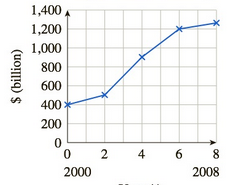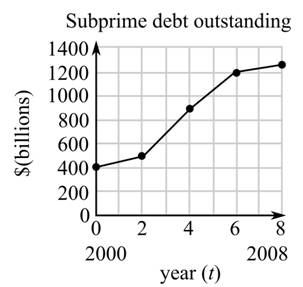Chapter 10.4, Problem 30EFinite Mathematics and Applied Cal...

7th Edition
Stefan Waner + 1 other
ISBN: 9781337274203

Solutions

Chapter
SectionFinite Mathematics and Applied Cal...

7th Edition
Stefan Waner + 1 other
ISBN: 9781337274203
Textbook Problem

Subprime Mortgage Debt during the Housing Crisis The following graph shows the approximate value V ( t ) ofsubprime (normally classified as risky) mortgage debt outstanding in the United States:37Subprime debt outstandingYear (t)a. Use the graph to estimate, to one decimal place, the average rate of change of V ( t ) withrespect to t over the interval | 2 , 6 | , and interpret the result.b. Over which 2-year period(s) was the average rate of change of V ( t ) theleast? [HINT: See Example 2.]

(a)

To determine

To calculate: The average rate of change of V(t) over the interval [2,6], if the graph of the percentage P(t) of mortgages debt outstanding in the United States with respect to years is shown below.Explanation

Given Information:

The provided interval is [2,6] and the graph of the value V(t) of mortgages debt outstanding in the United States with respect to years is shown below:

Formula used:

The average rate of change f(x) over the interval [a,b] is:

Average rate of change of f=Change in fChange in x=ΔfΔx=f(b)f(a)ba

Calculation:

Consider the interval [2,6].

Refer the provided graph and find the value of V(t) corresponding to interval [2,6]

(b)

To determine

The two year period at which the average rate of change V(t) was least, if the graph of the percentage P(t) of mortgages debt outstanding in the United States with respect to years is shown below.Still sussing out bartleby?

Check out a sample textbook solution.

See a sample solution

The Solution to Your Study Problems

Bartleby provides explanations to thousands of textbook problems written by our experts, many with advanced degrees!

Get Started

In Exercises 16, simplify the expression. 6. (1+x2)2(2)2x(2)(1+x2)(2x)(1+x2)4

Applied Calculus for the Managerial, Life, and Social Sciences: A Brief Approach

Simplify: 14x256x228x7x

Elementary Technical Mathematics

True or False: The slope of a tangent line may be interpreted as average velocity.

Study Guide for Stewart's Single Variable Calculus: Early Transcendentals, 8th

True or False: If R is a rectangular region in the plane, then

Study Guide for Stewart's Multivariable Calculus, 8th

In Exercises 5-8, find the values of the variables. [1xy3]=[z23w]

Finite Mathematics for the Managerial, Life, and Social Sciences